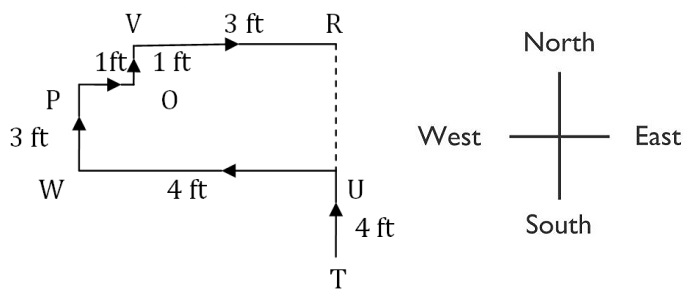## Direction and Distance

#### Direction and Distance

1. Going 50 m to the South of her house, Radhika turns left and goes another 20 m. Then, turning to the North, she goes 30 m and then starts walking to her house. In which direction is she walking now?

1. Draw a figure with the movement of Radhika with the help of direction figure given below.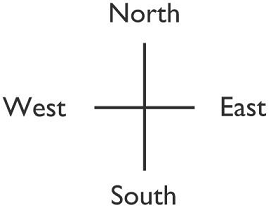##### Correct Option: A

Let us assume her house is situated at point A.
Draw a figure of movement of Radhika.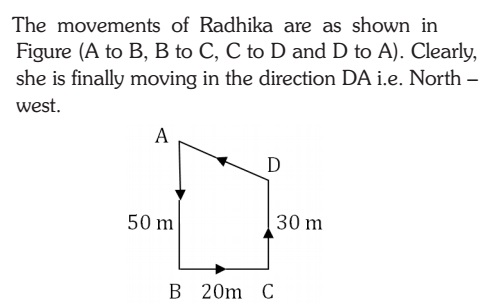1. I am facing east. I turn 100° in the clockwise direction and then 145° in the anti clock wise direction. Which direction am I facing now?

1. Draw a figure, in which a man standing in East direction.##### Correct Option: B

Draw a figure first when man is moving in clockwise direction then for anticlockwise. The man will be finally standing in North East position as explain in below figure.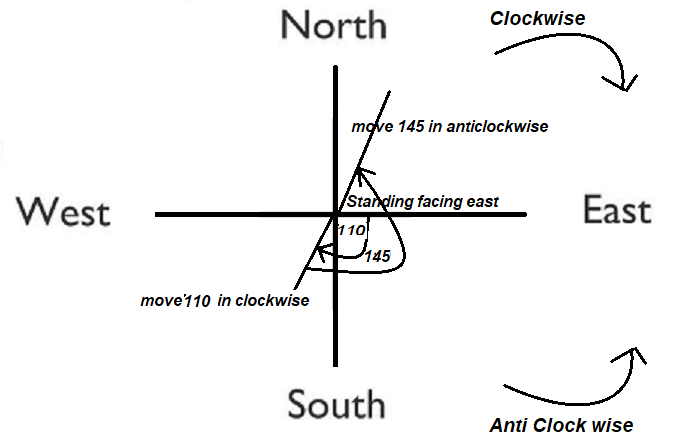1. You go north, turn right, then right again and then go to the left. In which direction are you now?

1. First move to north direction, then turn right, again turn right without moving from the position than turn left and move.##### Correct Option: C

First move to north direction, then turn right, again turn right without moving from the position than turn left and move.1. Rohan walks a distance of 3 km towards North, then turns to his left and walks for 2 km. He again turns left and walks for 3 km. At this point he turns to his left and walks for 3 km. How many kilometers is he from the starting point?

1. Draw a figure as per given question.##### Correct Option: A

Draw a figure.
Rohan walks a distance of 3 km towards North.
Then turns to his left and walks for 2 km.
He again turns left and walks for 3 km.
At this point he turns to his left and walks for 3 km.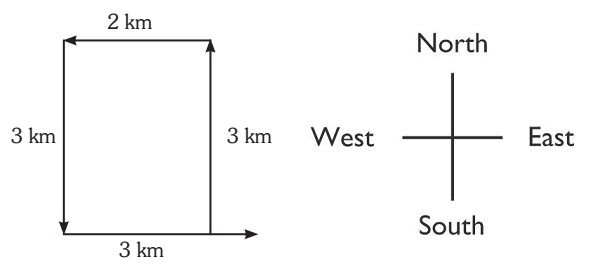1. Maya starts at point T, Walks straight to point U which is 4 ft away. She turns left at 90° and walks to W which is 4 ft away, turns 90° right and goes 3 ft to P, turns 90° right and walks 1 ft to Q, turns left at 90° and goes to V, which is 1 ft away and once again turns 90° right and goes to R, 3 ft away. What is the distance between T and R?

1. Draw a figure for the movement of maya with the help of direction figure which is given below.##### Correct Option: D

The movements of Maya from T to R are as shown in Figure.
Distance between T and R = TR = TU + UR = TU + PW + QV = ( 4 + 3 + 1) ft = 8 ft.